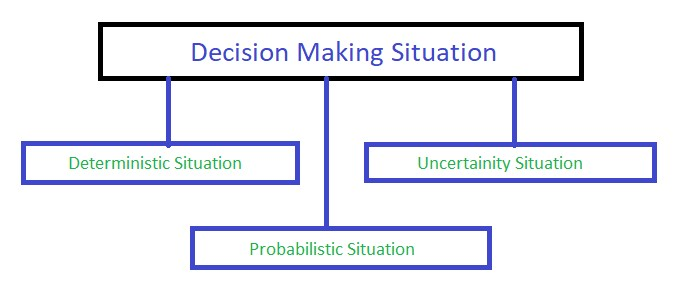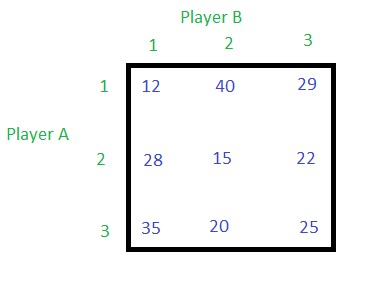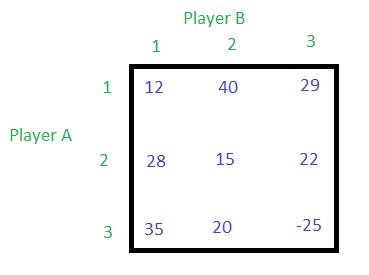# Game Theory (Normal – form game) | Set 1 (Introduction)

• Difficulty Level : Expert
• Last Updated : 02 Aug, 2019

Game theory is a mathematical model used for decision making. It has applications in all fields of social science, as well as in logic and computer science. Game theory has come to play an increasingly important role in logic and in computer science. To be fully defined, a game must specify the following elements: the players of the game, the information and actions available to each player at each decision point, and the payoffs for each outcome. Most cooperative games are presented in the characteristic function form, while the extensive and the normal forms are used to define non-cooperative games.

In game theory, normal form or it is also called strategic form , is a description of a game. The normal (or strategic form) game is usually represented by a matrix which shows the players, strategies, and payoffs. When a game is presented in normal form, it is presumed that each player acts simultaneously or, at least, without knowing the actions of the other.

Decision Making Situation
Generally, decision making situation can be classified into three different categories as shown in the figure below:Basic Terminologies of Game Theory

1. Players : Generally there are two players in a game. For example, Player A and Player B, or Company A and Company B.
2. Strategies : Strategy means it is a course of action taken by a player, for example, a company will have different strategies to increase the volumes of sales. Generally, in-game theory there are two strategies, the first one pure strategy, and the second one mixed strategy. If a company selects only one particular strategy leaving the remaining strategy then it is said as pure strategy, but the sum of these probabilities is always equal to 1 (see example below). If the player follows more than one strategies, then the player is said to follow mixed strategy, and where the probability of selection of a particular or individual strategy is always less than one so that the sum of all the probabilities will be equal to 1 (see example below).

Example: Suppose there are three strategies S1, S2 and S3.

Pure strategy : When the player selects one strategy, lets say S2, then the probability of S2 becomes 1 and the remaining two strategy’s probability will be 0. Hence the total probability’s sum is 0 + 1 + 0 = 1.

Mixed strategy : When a player selects two strategies, let’s say S1 and S3, and their probabilities are given as 0.62 and 0.38 respectively, and the probability of strategy S2 is 0. Hence the total probability’s sum is 0.62 + 0 + 0.38 = 1.

3. Payoff Matrix : A sample payoff matrix is shown below. There are two players, player A and player B with three strategies each i.e. 1, 2 and 3. The inner values in the matrix is the outcome of different combinations. If player A selects the 3rd strategy and the player B selects the 1st strategy, then the outcome will be 35, and if the player A selects the 2nd strategy and the player B also selects the 2nd strategy then the outcome will be 15.If the outcome is positive, then it is a gain to player A and loss to player B. If the outcome is negative, then it is loss to player A and gain to player B. Consider the below payoff matrix, if the outcome is -25 then player A loses 25 points while player B gains 25 points.4. Maximin Principle : Maximizes the minimum guaranteed gains.
5. Minimax Principle : Minimizes the maximum losses.
6. Saddle Point : The game will have saddle point if maximin value and minimax value are equal i.e. the intersecting point will be equal. When there is no saddle point in the game then the game is said to have a mixed strategy.
7. Value of the Game : If the game has the saddle point, then the outcome in the cell at the saddle point is called the value of the game.
8. Two – Person Zero-sum game : In a game with two players, if the gain of one player is equal to the loss of another player, then that game is called a two-person zero-sum game. For better understanding see the above payoff matrix, if the outcome of the game is 40 then it is gain to the player A but loss to player B, and if the outcome is -25 then it is a loss to player A but gains to player B.
My Personal Notes arrow_drop_up